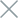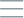• Home
• Tags
• Series
•# Linear Search Algorithm in C++ - Explanation, Example, and Code

The linear search algorithm is a simple method used to find a specific element in a list. This algorithm works by sequentially checking each element in the list until the desired element is found or the entire list is traversed.

## How It Works

1. Start from the first element in the list.
2. Compare the current element with the target value.
3. If the current element equals the target value, the algorithm terminates and returns the position of the element.
4. If not, continue iterating through the remaining elements in the list.
5. If the entire list is traversed without finding the target element, the algorithm returns a value indicating not found.

## Example

Let's say we have a list of integers and we want to find the number 34 in the list.

List: {12, 45, 67, 89, 34, 56, 23, 90}

1. Start at the first element: 12. Not the desired number.
2. Move to the next element: 45. Not the desired number.
3. Continue with the remaining elements: 67, 89, 34. Element 34 matches the desired number.
4. The algorithm terminates and returns the position of 34, which is 4.

## Example Code in C++

``````#include <iostream>
#include <vector>

int linearSearch(const std::vector<int>& arr, int target) {
for (int i = 0; i < arr.size(); ++i) {
if (arr[i] == target) {
return i;
}
}
return -1;
}

int main() {
std::vector<int> numbers = {12, 45, 67, 89, 34, 56, 23, 90};
int target = 34;

int result = linearSearch(numbers, target);

if (result != -1) {
std::cout << "Element " << target << " found at position " << result << std::endl;
} else {
std::cout << "Element " << target << " not found in the array" << std::endl;
}

return 0;
}
``````

In the given example, we've used the `linearSearch` function to find the number 34 in the list of integers. The result will be the position of 34 in the list (positions start from 0) or -1 if the number is not found.